.NET Framework 4.5.2+

# RowCollection Interface

A collection of all rows in a worksheet.

## Declaration

``public interface RowCollection``

## Remarks

Use the RowCollection object’s members to access, add, hide, group, and remove rows.

### Access Rows

Use the RowCollection.Item property to access an individual row in a worksheet.

#### Obtain the Row at the Specified Index

``````using DevExpress.Spreadsheet;
// ...

// Access the row collection.
RowCollection rows = workbook.Worksheets.Rows;

// Access the first row in the collection.
Row firstRow = rows;
``````

A row index is zero-based. It specifies the row position in the collection.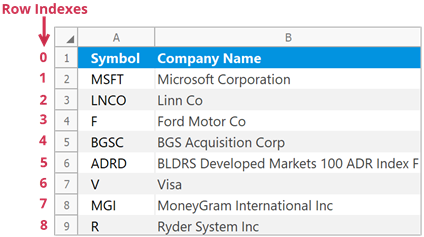#### Obtain the Row with a Given Heading

``````using DevExpress.Spreadsheet;
// ...

// Access the row collection.
RowCollection rows = workbook.Worksheets.Rows;

// Access the first row by its heading.
Row firstRow = rows["1"];
``````

Row headings help users identify each row in a worksheet.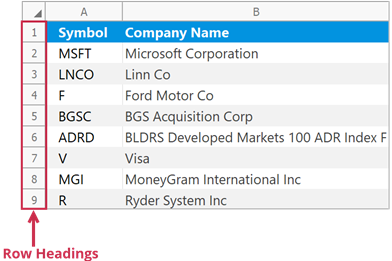### Insert Rows

Use the following methods to insert rows into a worksheet:

Row.Insert
Inserts a row above the current row.
RowCollection.Insert
Inserts rows at the specified position.

Note

The number of rows in a worksheet does not change - 1,048,576. When you add new rows, the rows below are shifted down and an equivalent number of rows at the end of the worksheet is removed.

#### Insert a Single Row

``````// Insert the third row.
worksheet.Rows["3"].Insert();

// Insert the fifth row.
worksheet.Rows.Insert(4);
``````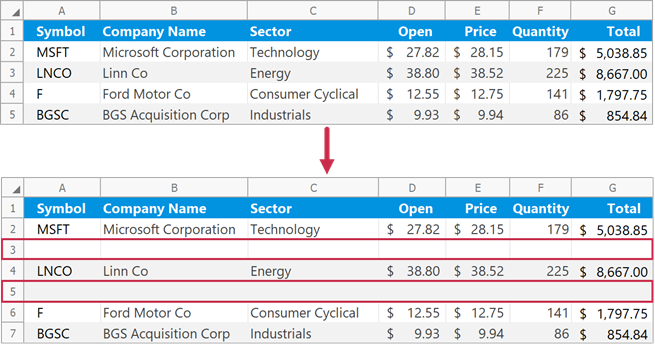#### Insert Multiple Rows

``````// Insert three rows (from row 3 to row 5).
worksheet.Rows.Insert(2, 3);
``````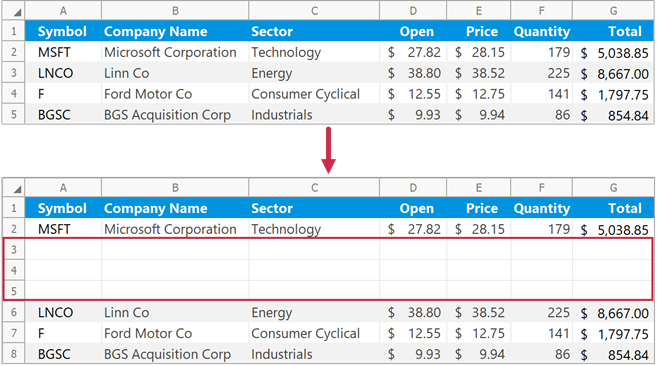#### Insert Rows Above a Specific Cell Range

Use the Worksheet.InsertCells method to insert empty rows above the specified cells. The method inserts the same number of rows as the specified cell range.

``````// Insert two rows above the "B3:E4" cell range.
worksheet.InsertCells(worksheet.Range["B3:E4"], InsertCellsMode.EntireRow);
``````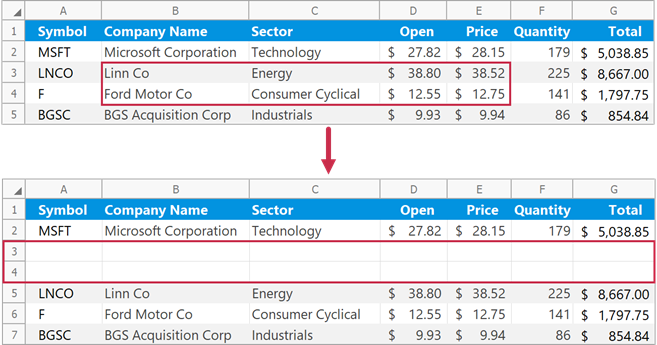#### Format Inserted Rows

Pass a RowFormatMode enumeration member to the RowCollection.Insert method to specify format options for inserted rows.

``````// Insert two rows with the same formatting as the first row.
worksheet.Rows.Insert(1, 2, RowFormatMode.FormatAsPrevious);
``````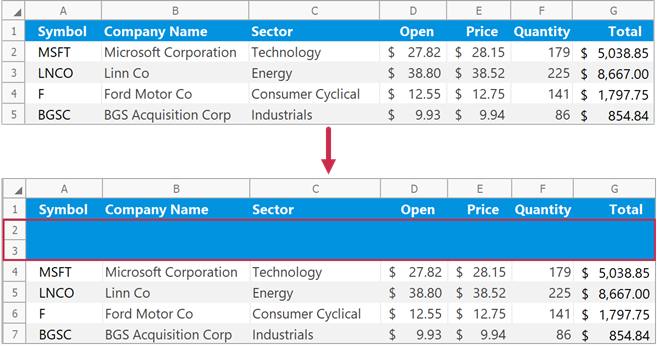### Remove Rows

Use the following methods to delete rows from a worksheet:

Row.Delete
Removes the current row.
RowCollection.Remove
Removes rows at the specified position.

Note

The number of rows in a worksheet does not change - 1,048,576. When you delete rows, the rows below are shifted up and an equivalent number of new rows is added to the end of the worksheet.

#### Remove a Single Row

``````// Delete the second row.
worksheet.Rows.Delete();

// Delete the third row.
worksheet.Rows.Remove(2);
``````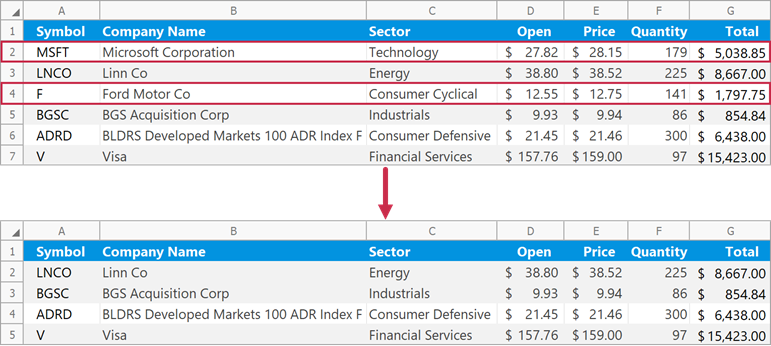#### Remove Multiple Rows

``````// Delete three rows (from row 2 to row 4).
worksheet.Rows.Remove(1, 3);
``````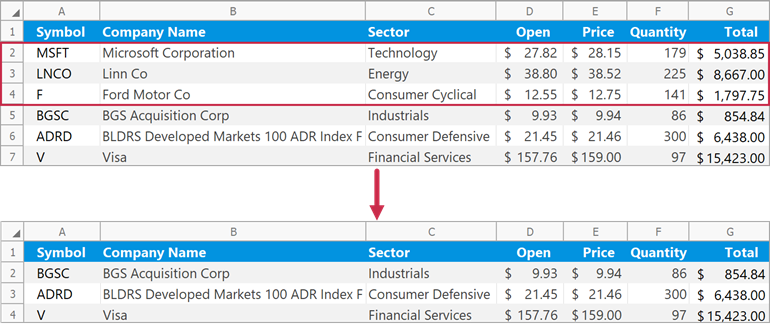#### Remove Rows That Meet a Specific Condition

``````// Specify the condition to remove worksheet rows.
// If a value in the "D" column is greater than 30, remove the corresponding row.
Func<int, bool> rowRemovalCondition = x => worksheet.Cells[x, 3].Value.NumericValue > 30.0;

// Delete rows that meet the specified condition.
// Check rows 2 through 7.
worksheet.Rows.Remove(1, 6, rowRemovalCondition);
``````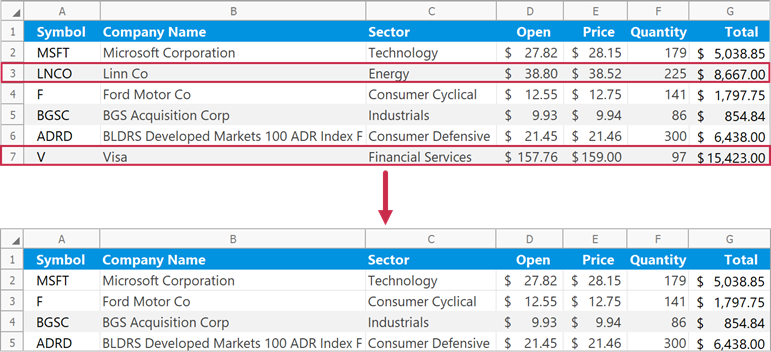#### Remove Rows That Contain Specific Cells

Use the Worksheet.DeleteCells method to delete rows that contain the specified cell range.

``````// Delete a row that contains the "B2" cell.
worksheet.DeleteCells(worksheet.Cells["B2"], DeleteMode.EntireRow);
``````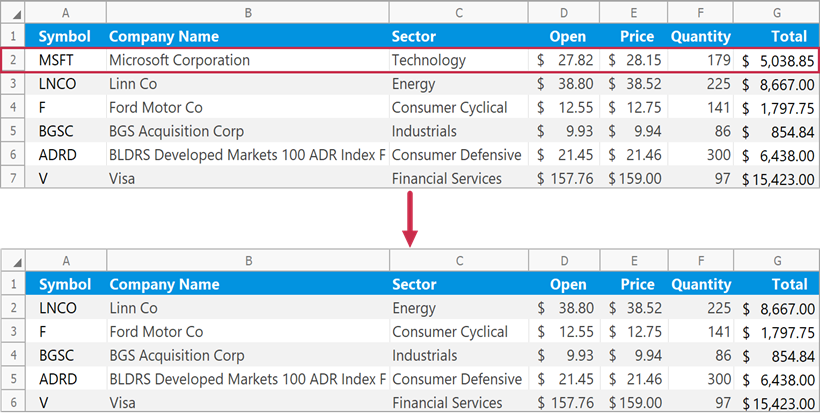### Group and Ungroup Rows

Use the RowCollection.Group method to group rows.

``````// Group rows 3 through 6 and collapse the group.
worksheet.Rows.Group(2, 5, true);

// Group rows 9 through 12 and expand the group.
worksheet.Rows.Group(8, 11, false);

// Group rows 2 through 13 to create the outer group.
worksheet.Rows.Group(1, 12, false);
``````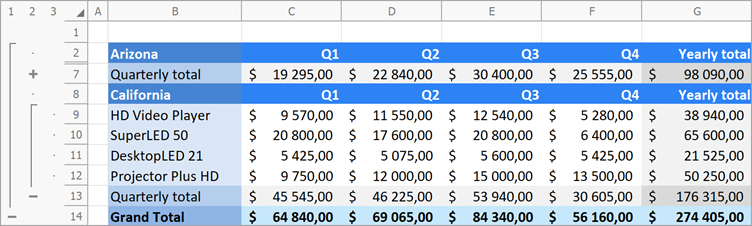Use the RowCollection.UnGroup method to ungroup rows.

``````// Ungroup rows 3 through 6 and display collapsed data.
worksheet.Rows.UnGroup(2, 5, true);

// Ungroup rows 9 through 12.
worksheet.Rows.UnGroup(8, 11, false);

// Remove the outer row group.
worksheet.Rows.UnGroup(1, 12, false);
``````

#### Expand or Collapse Groups

Use the following methods to collapse or expand row groups in a worksheet:

RowCollection.CollapseAllGroups
Collapses all row groups.
RowCollection.CollapseGroups
Collapses row groups starting with the specified group level.
RowCollection.ExpandAllGroups
Expands all row groups.
``````// Collapse all row groups except the first level group.
worksheet.Rows.CollapseGroups(2);
``````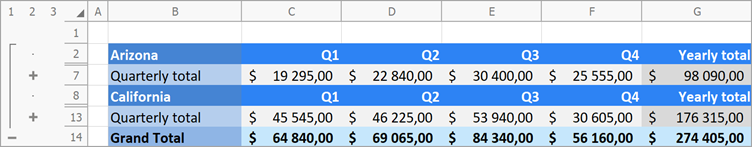### Hide Rows

Use the Row.Visible property or the RowCollection.Hide method to hide rows in a worksheet.

``````// Hide rows 2 through 6.
worksheet.Rows.Hide(1, 5);

// Hide row 8.
worksheet.Rows.Visible = false;
``````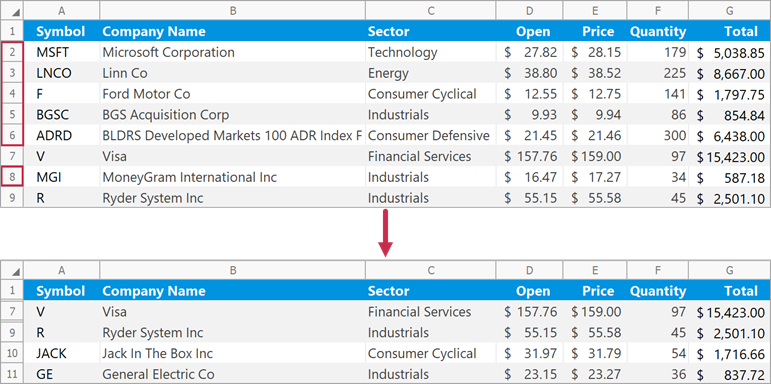Set the Row.Visible property to true to display a row. Use the RowCollection.Unhide method to show multiple hidden rows.

``````// Show rows 2 through 6.
worksheet.Rows.Unhide(1, 5);

// Show row 8.
worksheet.Rows.Visible = true;
``````